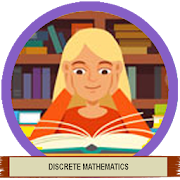# Learn Discrete Mathematics FullEveryone
10
Discrete Mathematics is a branch of mathematics involving discrete elements that uses algebra and arithmetic. It is increasingly being applied in the practical fields of mathematics and computer science. It is a very good tool for improving reasoning and problem-solving capabilities. This Learn Discrete Mathematics Full explains the fundamental concepts of Sets, Relations and Functions, Mathematical Logic, Group theory, Counting Theory, Probability, Mathematical Induction and Recurrence Relations, Graph Theory, Trees and Boolean Algebra.

This Learn Discrete Mathematics Full has been prepared for students pursuing a degree in any field of computer science and mathematics. It endeavors to help students grasp the essential concepts of discrete mathematics.

This Learn Discrete Mathematics Full has an ample amount of both theory and mathematics. The readers are expected to have a reasonably good understanding of elementary algebra and arithmetic.
Collapse

Review Policy
3.5
10 total
5
4
3
2
1

## What's New

Characteristics of the Learn Discrete Mathematics Full:
+ Free Book Apps.
+ Easy to use.
+ Have a list of related applications.
+ Easily view the history of viewed items.
+ Easily share with friends through all social networking channels.
Collapse

Updated
July 1, 2018
Size
9.6M
Installs
1,000+
Current Version
1.0
Requires Android
4.0 and up
Content Rating
Everyone
Permissions
Offered By